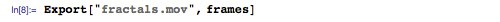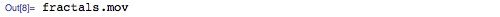Browse by Topic
Related Topics

# Mathematica Q&A: Creating Movie Files

Got questions about Mathematica? The Wolfram Blog has answers! We’ll regularly answer selected questions from users around the web. You can submit your question directly to the Q&A Team using this form.

Here is this week’s question:

How can I create and export movies and animations in Mathematica?

This is something we do often—just about every movie or animation on the Wolfram Blog is created in Mathematica.

If you are creating a movie that you want to include in a web page or send to someone as a standalone file, then the easiest and most interactive option is probably to distribute the movie in Computable Document Format (CDF). To do this, create an interactive animation using a function like Animate or ListAnimate: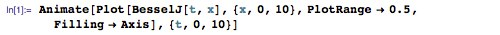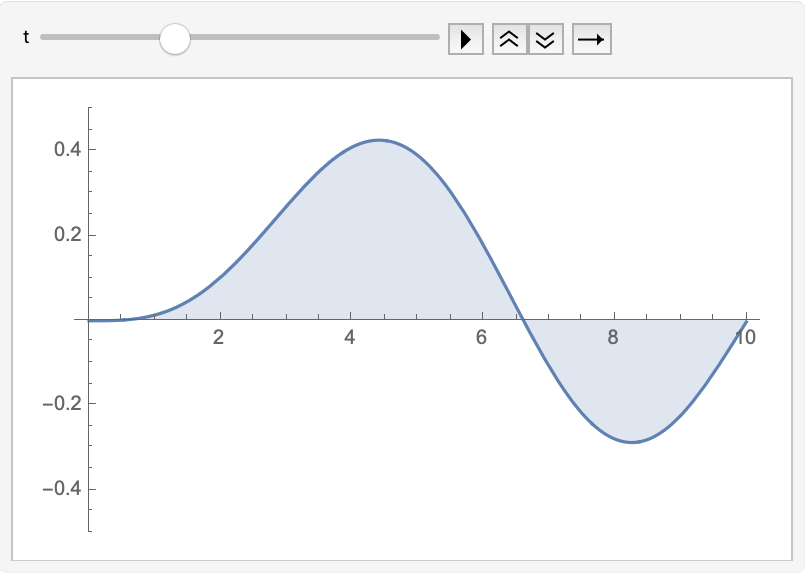The resulting animation can be saved as a CDF (File -> Save As -> Computable Document (.cdf)) and embedded live in a web page or distributed like any other file. Anyone with the Wolfram CDF Player can open and interact with the animation. The unique advantage of CDF is that you can very easily include additional interactive elements with your animation, such as controls generated by Manipulate: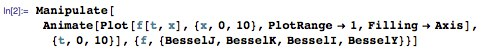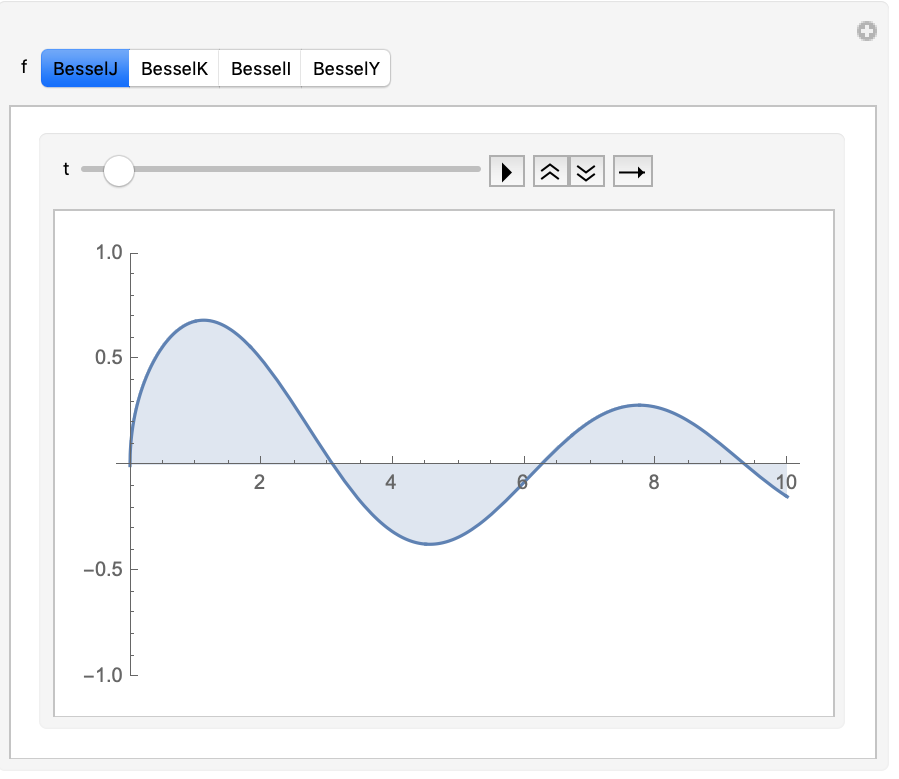If you want to create a regular movie in a file format such as QuickTime, then the first step is to prepare each frame of the animation. Here’s a function frame[t] generating one frame of animation at time t: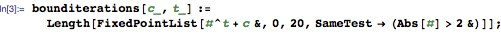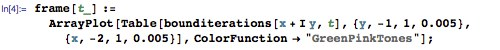The function bounditerations[c, t] counts the number of times that repeated applications of the complex function zz^t + c (starting from z = 0) stay inside the region |z|<2. The function frame[t] visualizes this count as a function of the complex parameter c: the horizontal axis is Re[c] and the vertical axis is Im[c].

When t = 2, you’ll probably recognize the result as the famous Mandelbrot set: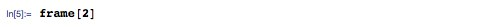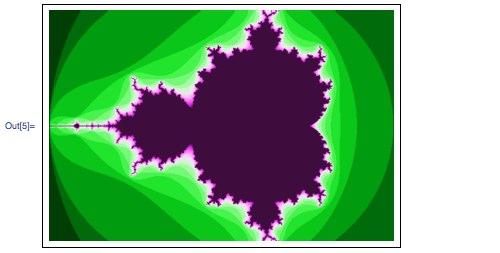Different values of t give interesting visualizations of other sets: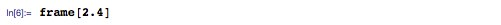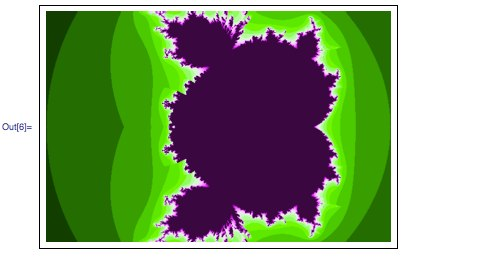You can animate this visualization by building up a set of frames, say from t = 2 to t = 4: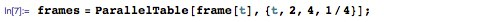(That line takes a few minutes to run on my computer. You could make it faster by taking advantage of new C compilation techniques in Mathematica 8.)

Once you have the list of frames, a simple Export command suffices to create the movie: